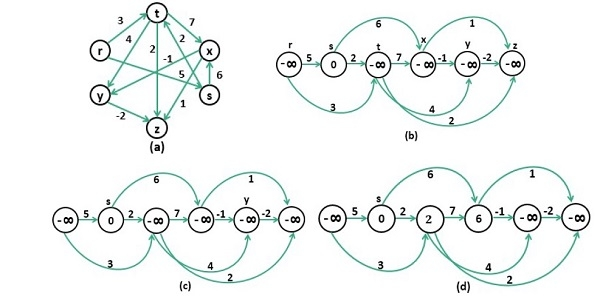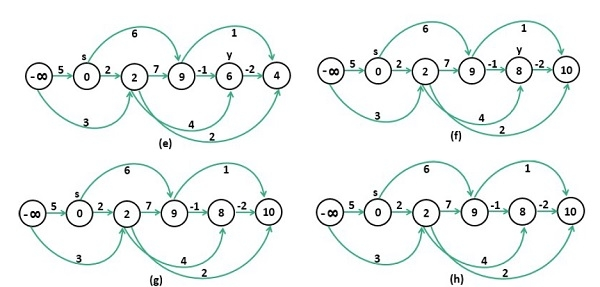# Longest Path in a Directed Acyclic Graph

One weighted directed acyclic graph is given. Another source vertex is also provided. Now we have to find the longest distance from the starting node to all other vertices, in the graph.We need to sort the nodes in topological sorting technique, and the result after the topological sort is stored into a stack. After that repeatedly popped from the stack and try to find the longest distance for each vertex.

## Input and Output

Input:
The cost matrix of the graph.
0  5   3 -∞ -∞ -∞
-∞ 0   2  6 -∞ -∞
-∞ -∞  0  7  4  2
-∞ -∞ -∞  0 -1  1
-∞ -∞ -∞ -∞  0 -2
-∞ -∞ -∞ -∞ -∞  0

Output:
Longest Distance from Source Vertex 1
Infinity 0 2 9 8 10

## Algorithm

topoSort(u, visited, stack)

Input: starting node u, the visited list to keep track, the stack.

Output − Sort the nodes in a topological way.

Begin
mark u as visited
for all vertex v, which is connected with u, do
if v is not visited, then
topoSort(v, visited, stack)
done
push u into the stack
End

longestPath(start)

Input − The starting node.

Output − List of longest distance of all vertices from the starting node.

Begin
initially make all nodes as unvisited
for each node i, in the graph, do
if i is not visited, then
topoSort(i, visited, stack)
done

make distance of all vertices as - ∞
dist[start] := 0
while stack is not empty, do
pop stack item and take into nextVert
if dist[nextVert] ≠ - ∞, then
for each vertices v, which is adjacent with nextVert, do
if cost[nextVert, v] ≠ - ∞, then
if dist[v] < dist[nectVert] + cost[nextVert, v], then
dist[v] := dist[nectVert] + cost[nextVert, v]
done
done

for all vertices i in the graph, do
if dist[i] = - ∞, then
display Infinity
else
display dist[i]
done
End

## Example

#include<iostream>
#include<stack>
#define NODE 6
#define INF -9999
using namespace std;

int cost[NODE][NODE] = {
{0, 5, 3, INF, INF, INF},
{INF, 0, 2, 6, INF, INF},
{INF, INF, 0, 7, 4, 2},
{INF, INF, INF, 0, -1, 1},
{INF, INF, INF, INF, 0, -2},
{INF, INF, INF, INF, INF, 0}
};

void topoSort(int u, bool visited[], stack<int>&stk) {
visited[u] = true;    //set as the node v is visited

for(int v = 0; v<NODE; v++) {
if(cost[u][v]) {    //for allvertices v adjacent to u
if(!visited[v])
topoSort(v, visited, stk);
}
}

stk.push(u);    //push starting vertex into the stack
}

void longestPath(int start) {
stack<int> stk;
int dist[NODE];
bool vis[NODE];

for(int i = 0; i<NODE;i++)
vis[i] = false;    // make all nodes as unvisited at first

for(int i = 0; i<NODE; i++)    //perform topological sort for vertices
if(!vis[i])
topoSort(i, vis, stk);

for(int i = 0; i<NODE; i++)
dist[i] = INF;    //initially all distances are infinity
dist[start] = 0;    //distance for start vertex is 0

while(!stk.empty()) {    //when stack contains element, process in topological order
int nextVert = stk.top(); stk.pop();

if(dist[nextVert] != INF) {
for(int v = 0; v<NODE; v++) {
if(cost[nextVert][v] && cost[nextVert][v] != INF) {
if(dist[v] < dist[nextVert] + cost[nextVert][v])
dist[v] = dist[nextVert] + cost[nextVert][v];
}
}
}
}

for(int i = 0; i<NODE; i++)
(dist[i] == INF)?cout << "Infinity ":cout << dist[i]<<" ";
}

main() {
int start = 1;
cout << "Longest Distance From Source Vertex "<<start<<endl;
longestPath(start);
}

## Output

Longest Distance From Source Vertex 1
Infinity 0 2 9 8 10